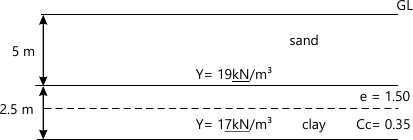MORE IN Geotechnical Engineering - 1
MU Civil Engineering (Semester 5)
Geotechnical Engineering - 1
May 2015
Total marks: --
Total time: --
INSTRUCTIONS
(1) Assume appropriate data and state your reasons
(2) Marks are given to the right of every question
(3) Draw neat diagrams wherever necessary

1 (a) What is geotechnical engineering? Explain the scope of soil engineering.
5 M
1 (b) Explain the Mohr-Coulomb theory for shearing strength of soils and its modifications by Terzaghi.
5 M
1 (c) What are the factors that influence permeability of soils?
5 M
1 (d) Differentiate compaction and consolidation?
5 M

2 (a) With soil mass idealization as three phase diagram and usual notations, derive from first principles that $S=\dfrac{w}{\dfrac{\gamma w}{\gamma}(1+w)-\dfrac{1}{G}}$
5 M
2 (b) A natural soil sample has a bulk density of 2g/cm3 with 6% water content. Calculate the amount of water required to be added to one cubic metre of soil to raise the water content to 15% while the void ratio remains constant. What is then the degree of saturation? Consider G=2.67.
10 M
2 (c) What are the factors affecting compaction of soil.
5 M

3 (a) Classify the following soil as per IS classification system systematically with justifications.
%passing 75 microns sieve=8%; retained on 4.75 sieve=35; coefficient of curvature=2.5; uniformity coefficient=7; liquid limit=15; plasticity index=3.
5 M
3 (b) A sample of soil for a constant head permeability test gives the following data; Diameter of permeameter=76 mm; length of soil sample =200 mm; head causing flow=15 mm; quantity of water collected in 10 minutes=150 ml; G=2.65; saturated unit weight of soil=20 kN/m3 determine (a) Coefficient of permeability of soil in cm/s(b) superficial velocity of flow (c) Seepage velocity.
10 M
3 (c) What are the used of consistency limits.
5 M

4 (a) Practically, under what circumstances quick sand conditions are developed. What is the critical hydraulic gradient for the quick sand condition in a soil deposit having porosity =33% and G=2.60.
5 M
4 (b) The water table in a deposit of uniform sand is located at 2m below the ground surface. Assuming the soil above the water table is dry, determine the effective stress at a depth of 5 m below the ground surface. The void ratio is 0.75 and specific gravity of solids is 2.65. If the soil above water table is saturated by capillary action, what is the effective stress at that depth?
10 M
4 (c) What is flow net? What are the characteristics of flow net?
5 M

5 (a) What is N value of standard penetration test used in soil exploration? Explain the various correction to be applied to the observed value of N. What is the importance of the test in geotechnical engineering?
10 M
5 (b) Calculate the final settlement of the clay layer shown in Figure 1 due to an increase of pressure of 30 kN/m2 at mid height of the layer. Also calculate the settlement when the water table rises to the ground surface.10 M

6 (a) Derive the relationship between the principle stresses at failure using Mohr-coulomb failure criteria $\sigma '_{1}=\sigma '_{3}N_{\varnothing }+2c'\sqrt{N_{\varnothing }}$
5 M
6 (b) In a direct shear test conducted on dense sand, the sample fails at a shear stress of 75 kN/m2 when the normal stress was held constant at 100 kN/m2. Draw the Mohr circle for the failure condition. Find (a) the angle of shearing resistance and (b) the magnitude and orientation of the major and minor principle stresses.
10 M
6 (c) Write a note on depth and spacing of bore holes. What are the salient features of bore log?
5 M

More question papers from Geotechnical Engineering - 1